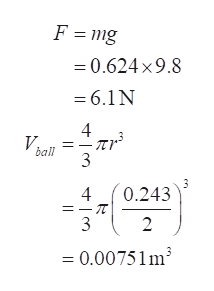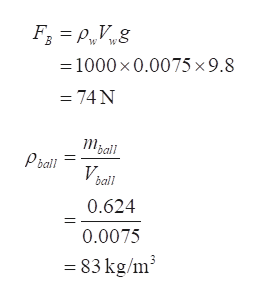# A standard basketball (mass = 624 grams with diameter of 24.3 cm) is held fully underwater. Calculate the bouyancy force and weight.A.) When released does the ball sink to the bottom or float to the surface?B.) If it floats, what percentage of the ball is sticking out of the water? If it sinks, what is the normal force with which is sits on the bottom of the pool?

Question
112 views

A standard basketball (mass = 624 grams with diameter of 24.3 cm) is held fully underwater. Calculate the bouyancy force and weight.

A.) When released does the ball sink to the bottom or float to the surface?

B.) If it floats, what percentage of the ball is sticking out of the water? If it sinks, what is the normal force with which is sits on the bottom of the pool?

check_circle

Step 1

Step 1

It is given that

Mass = 624 gm

Diameter = 24.3 cm

We need to find the buoyancy force and weight

Buoyant force is given as

Step 2

Step 2

The weight of the ball is

To find the buoyancy, we need the volume of displaced water, which is equivalent to the volume of the ball as it is completely submerged.help_outlineImage TranscriptioncloseF = mg = 0.624 x9.8 = 6.1N 4 V. ball 3 0.243 4 3 = 0.00751 m fullscreen
Step 3

Step 3

The buoyant force is then

Which is much stronger than the force due to gravity. That means, the ball will rise to the surfa...help_outlineImage TranscriptioncloseF; = P„V„g =1000 x 0.0075 ×9.8 = 74 N Mball Pball V. ball 0.624 0.0075 = 83 kg/m? fullscreen

### Want to see the full answer?

See Solution

#### Want to see this answer and more?

Solutions are written by subject experts who are available 24/7. Questions are typically answered within 1 hour.*

See Solution
*Response times may vary by subject and question.
Tagged in

### Fluid Mechanics